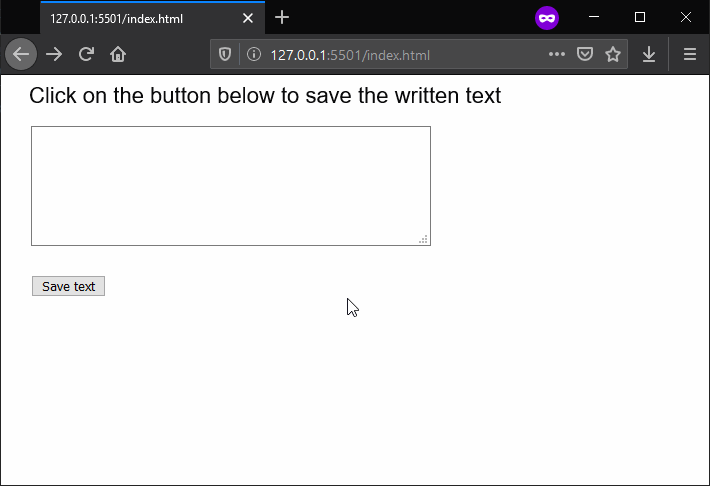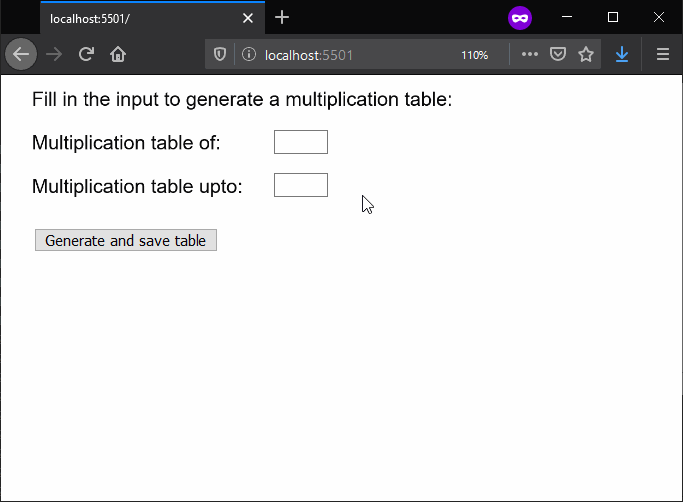# p5.js | saveStrings() Function

The saveStrings() function is used to write an array of strings with one line per string to the file. The saving of the file will vary depending on the web browser.

Syntax:

`saveStrings( list, filename, extension )`

Parameters: This function accept three parameter as mentioned above and described below:

• list: It is the array of strings that has to be written to the file.
• filename: It specifies the string that is used as the filename of the saved file.
• extension: It specifies the string that is used as the extension of the saved file. It is an optional parameter.

Below examples illustrate the saveStrings() function in p5.js:

Example 1:

 `function` `setup() { ` `    ``createCanvas(600, 300); ` `    ``textSize(22); ` `  `  `    ``// Create a textarea for ` `    ``// the input of text ` `    ``inputArea = createElement(``'textarea'``); ` `    ``inputArea.position(30, 50) ` `    ``inputArea.size(400, 120); ` `  `  `    ``// Create a button for saving text ` `    ``saveBtn = createButton(``"Save text"``); ` `    ``saveBtn.position(30, 200) ` `    ``saveBtn.mousePressed(saveFile); ` `} ` `  `  `function` `draw() { ` `    ``clear(); ` `    ``text(``"Click on the button below to "` `        ``+ ``"save the written text"``, 20, 20); ` `} ` `  `  `function` `saveFile() { ` `     `  `    ``// Get the value of the textarea ` `    ``// and split the strings on the basis ` `    ``// of the nextline character ` `    ``stringList = inputArea.value().split(``"\n"``); ` `  `  `    ``// Save the strings to file ` `    ``saveStrings(stringList, ``'output.txt'``); ` `} `

Output:Example 2:

 `function` `setup() { ` `    ``createCanvas(600, 300); ` `    ``textSize(18); ` `  `  `    ``// Create both inputs for the ` `    ``// multiplication table ` `    ``multiOf = createInput(); ` `    ``multiOf.position(250, 50) ` `    ``multiOf.size(50); ` `  `  `    ``multiTo = createInput(); ` `    ``multiTo.position(250, 90) ` `    ``multiTo.size(50); ` `  `  `    ``// Create a button for saving text ` `    ``saveBtn = createButton( ` `        ``"Generate and save table"``); ` `    ``saveBtn.position(30, 140) ` `    ``saveBtn.mousePressed(saveFile); ` `} ` `  `  `function` `draw() { ` `    ``clear(); ` `    ``text(``"Fill in the input to generate "` `        ``+ ``"a multiplication table:"``, 20, 20); ` `    ``text(``"Multiplication table of:"``, 20, 60); ` `    ``text(``"Multiplication table upto:"``, 20, 100); ` `} ` `  `  `function` `saveFile() { ` `     `  `    ``// Get the value of the two inputs ` `    ``// and generate table ` `    ``let mul = multiOf.value(); ` `    ``let maxUpto = multiTo.value(); ` `    ``let stringList = []; ` `  `  `    ``for` `(let i = 0; i < maxUpto; i++) { ` `        ``stringList[i] = mul + ``" * "` `            ``+ i + ``" = "` `+ mul * i; ` `    ``} ` `  `  `    ``// Save the strings to file ` `    ``saveStrings(stringList, ``'output.txt'``); ` `} `

Output:Online editor: https://editor.p5js.org/My Personal Notes arrow_drop_upCheck out this Author's contributed articles.

If you like GeeksforGeeks and would like to contribute, you can also write an article using contribute.geeksforgeeks.org or mail your article to contribute@geeksforgeeks.org. See your article appearing on the GeeksforGeeks main page and help other Geeks.

Please Improve this article if you find anything incorrect by clicking on the "Improve Article" button below.

Article Tags :

Be the First to upvote.

Please write to us at contribute@geeksforgeeks.org to report any issue with the above content.